## Oblate Spheroidal Coordinates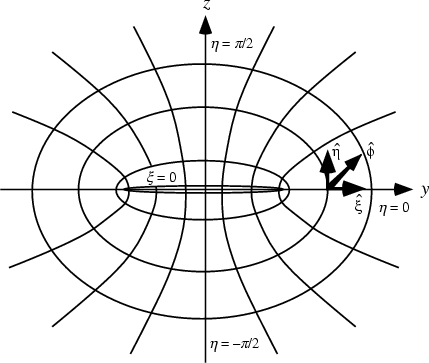A system of Curvilinear Coordinates in which two sets of coordinate surfaces are obtained by revolving the curves of the Elliptic Cylindrical Coordinates about the y-Axis which is relabeled the z-Axis. The third set of coordinates consists of planes passing through this axis.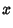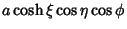(1)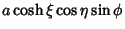(2)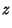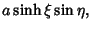(3)

where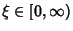,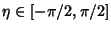, and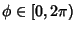. Arfken (1970) uses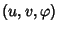instead of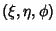. The Scale Factors are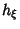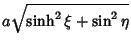(4)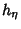(5)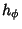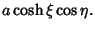(6)

The Laplacian is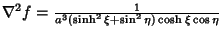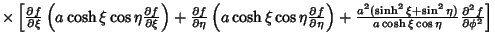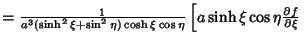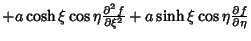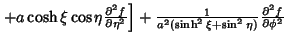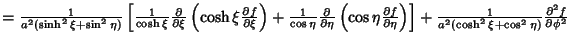(7)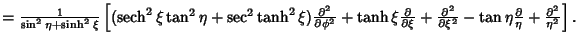(8)

An alternate form useful for two-center'' problems is defined by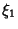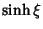(9)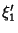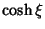(10)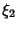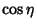(11)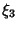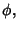(12)

where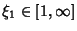,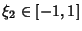, and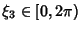. In these coordinates,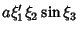(13)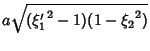(14)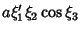(15)

(Abramowitz and Stegun 1972). The Scale Factors are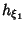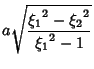(16)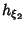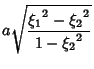(17)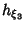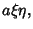(18)

and the Laplacian is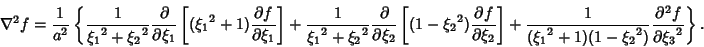(19)

The Helmholtz Differential Equation is separable.

See also Helmholtz Differential Equation--Oblate Spheroidal Coordinates, Latitude, Longitude, Prolate Spheroidal Coordinates, Spherical Coordinates

References

Abramowitz, M. and Stegun, C. A. (Eds.). Definition of Oblate Spheroidal Coordinates.'' §21.2 in Handbook of Mathematical Functions with Formulas, Graphs, and Mathematical Tables, 9th printing. New York: Dover, p. 752, 1972.

Arfken, G. Prolate Spheroidal Coordinates (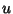,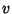,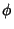).'' §2.11 in Mathematical Methods for Physicists, 2nd ed. Orlando, FL: Academic Press, pp. 107-109, 1970.

Morse, P. M. and Feshbach, H. Methods of Theoretical Physics, Part I. New York: McGraw-Hill, p. 663, 1953.